2020/12/10 13:11下载安装命令

## CPU版本安装命令

## GPU版本安装命令
pip install -f https://paddlepaddle.org.cn/pip/oschina/gpu paddlepaddle-gpu

## 目录：

2. 数据集简介；
3. 定义数据加载器；
4. 定义并训练模型；
5. 评估模型性能；
7. 总结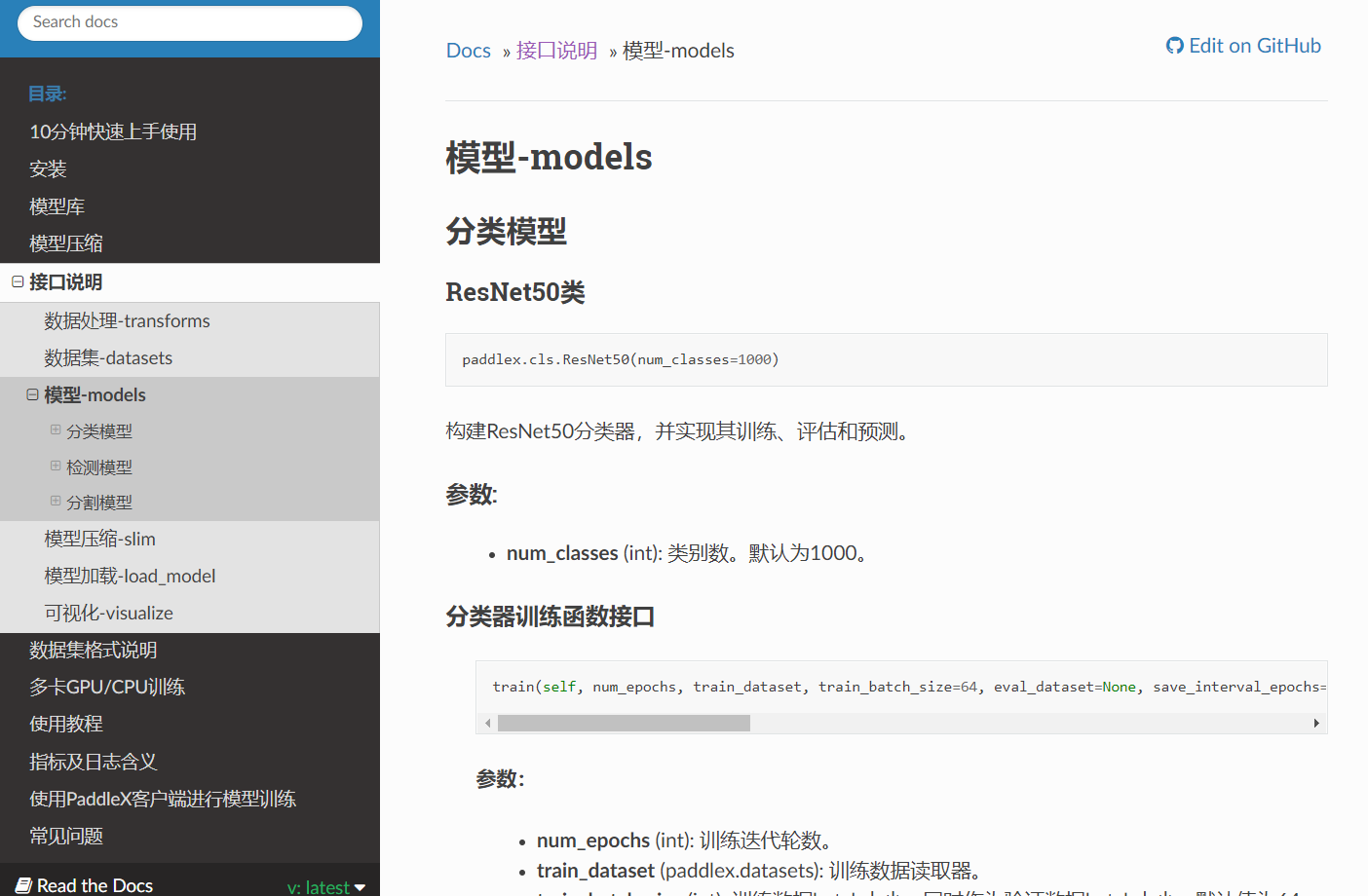### 二、数据集简介：

    'c0': 'normal driving',

'c1': 'texting-right',

'c2': 'talking on the phone-right',

'c3': 'texting-left',

'c4': 'talking on the phone-left',

'c6': 'drinking',

'c7': 'reaching behind',

'c8': 'hair and makeup',

'c9': 'talking to passenger'


#解压数据集
# !unzip /home/aistudio/data/data35503/imgs.zip -d /home/aistudio/work/imgs
# !cp /home/aistudio/data/data35503/lbls.csv /home/aistudio/work/

!pip install paddlex -i https://mirror.baidu.com/pypi/simple
!pip install paddlepaddle-gpu==1.7.0.post107 -i https://mirror.baidu.com/pypi/simple
import os
# 设置使用0号GPU卡（如无GPU，执行此代码后仍然会使用CPU训练模型）
os.environ['CUDA_VISIBLE_DEVICES'] = '0'
os.chdir('/home/aistudio/work/')
# jupyter中使用paddlex需要设置matplotlib
import matplotlib
matplotlib.use('Agg')
import paddlex as pdx

### 三、定义数据加载器：

import paddlehub as hub
import numpy as np

base = './data/'

datas = []
for i in range(10):
c_base = base+'train/c{}/'.format(i)
for im in os.listdir(c_base):
pt = os.path.join('train/c{}/'.format(i), im)
line = '{} {}'.format(pt, i)
# print(line)
datas.append(line)

np.random.seed(10)
np.random.shuffle(datas)

total_num = len(datas)
train_num = int(0.8*total_num)
test_num = int(0.1*total_num)
valid_num = total_num - train_num - test_num

print('train:', train_num)
print('valid:', valid_num)
print('test:', test_num)

with open(base+'train_list.txt', 'w') as f:
for v in datas[:train_num]:
f.write(v+'\n')

with open(base+'test_list.txt', 'w') as f:
for v in datas[-test_num:]:
f.write(v+'\n')

with open(base+'val_list.txt', 'w') as f:
for v in datas[train_num:-test_num]:
f.write(v+'\n')
train: 17939
valid: 2243
test: 2242


from paddlex.cls import transforms
train_transforms = transforms.Compose([
transforms.RandomCrop(crop_size=224),
transforms.RandomHorizontalFlip(),
transforms.Normalize()
])
eval_transforms = transforms.Compose([
transforms.ResizeByShort(short_size=256),
transforms.CenterCrop(crop_size=224),
transforms.Normalize()
])
train_dataset = pdx.datasets.ImageNet(
data_dir='data',
file_list='data/train_list.txt',
label_list='data/labels.txt',
transforms=train_transforms,
shuffle=True)
eval_dataset = pdx.datasets.ImageNet(
data_dir='data',
file_list='data/val_list.txt',
label_list='data/labels.txt',
transforms=eval_transforms)
2020-05-18 07:57:03 [INFO]	Starting to read file list from dataset...
2020-05-18 07:57:03 [INFO]	17939 samples in file data/train_list.txt
2020-05-18 07:57:03 [INFO]	Starting to read file list from dataset...
2020-05-18 07:57:03 [INFO]	2243 samples in file data/val_list.txt


num_classes = len(train_dataset.labels)
print(num_classes)
10


## 四、定义并训练模型：

MobileNetv3详细介绍可以看我的这一篇博客：

https://blog.csdn.net/weixin_44936889/article/details/104243853### MobileNet简介：

MobileNet 提出了使用深度分离卷积减少参数的方法；

#### DW Conv：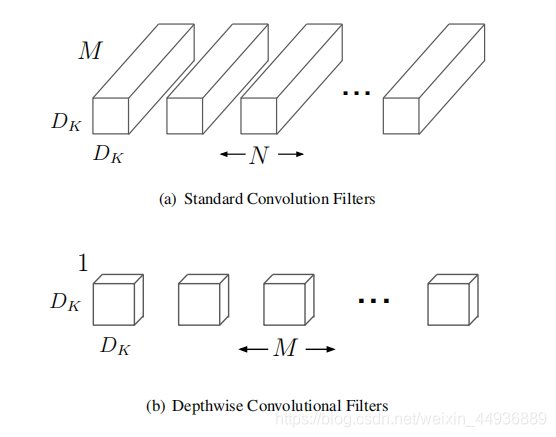### （一）论文地址：

《Searching for MobileNet V3》

### （二）核心思想：

2. 提出了一个对于移动设备更适用的非线性函数 h − s w i s h [ x ] = x R e L U 6 ( x + 3 ) 6 h-swish[x]=x\frac{ReLU6(x+3)}{6} hswish[x]=x6ReLU6(x+3)
3. 提出了 M o b i l e N e t V 3 − L a r g e MobileNetV3-Large MobileNetV3Large M o b i l e N e t V 3 − S m a l l MobileNetV3-Small MobileNetV3Small 两个新的高效率网络；
4. 提出了一个新的高效分割（指像素级操作，如语义分割）的解码器（ d e c o d e r decoder decoder）；

### （三）Platform-Aware NAS for Block-wise Search：

#### 3.1 MobileNetV3-Small：

A C C ( m ) × [ L A T ( m ) / T A R ] w ACC(m)×[LAT(m)/TAR]^w ACC(m)×[LAT(m)/TAR]w

1. 生成一系列建议模型（proposals），每个建议模型代表了一种结构改进，满足延迟至少比上一步的模型减小了 δ \delta δ，其中 δ = 0.01 ∣ L ∣ \delta=0.01|L| δ=0.01L L L L 是种子模型的延迟；
2. 对于每一个建议模型，使用上一步的预训练模型，删除并随机初始化改进后丢失的权重，继续训练 T T T 步来粗略估计建议模型的准确率，其中 T = 10000 T=10000 T=10000
3. 根据某种度量，选取最合适的建议模型，直到达到了目标延迟 T A R TAR TAR

1. 减小 Expansion Layer 的大小；
2. 同时减小 BottleNeck 模块中的前后残差项的 channel 数；

### （五）Efficient Mobile Building Blocks：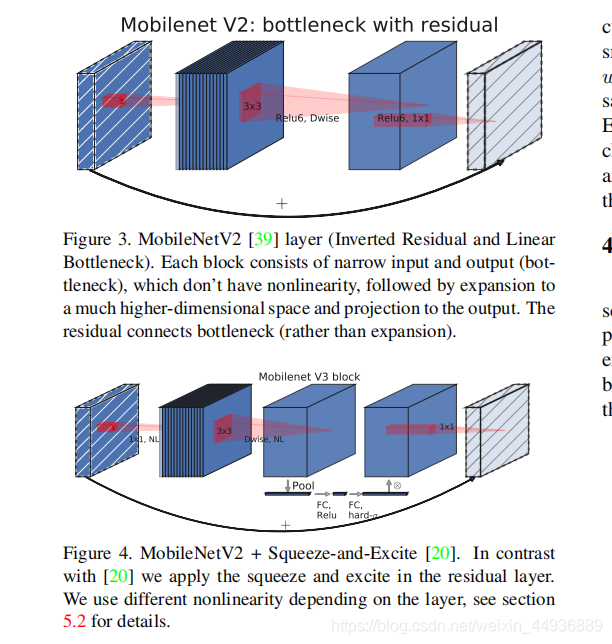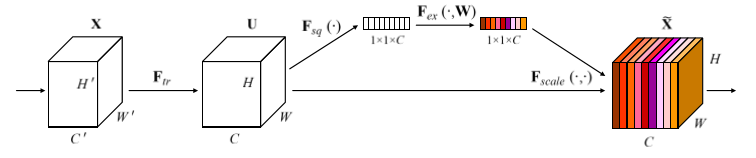### （六）Redesigning Expensive Layers：

#### 6.1 Last Stage：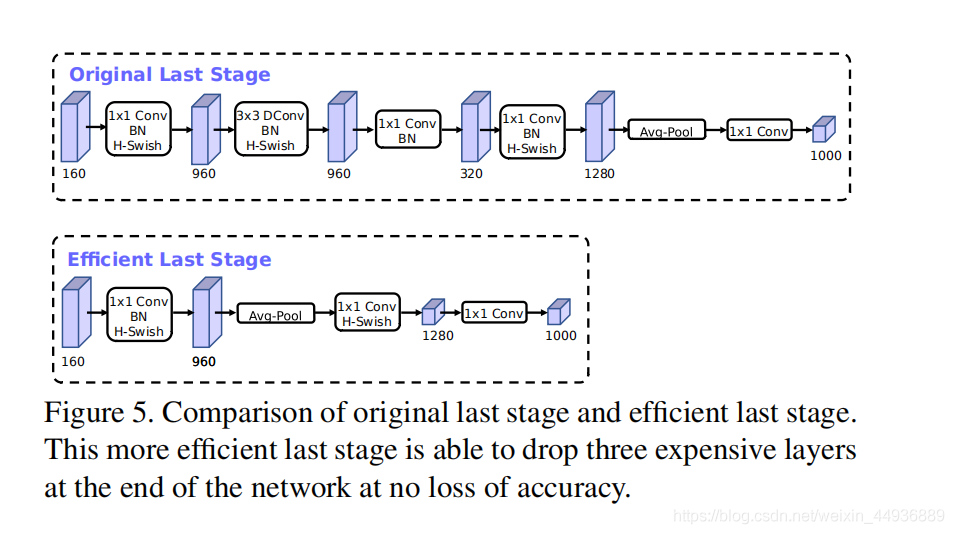### （七）hard switch 函数：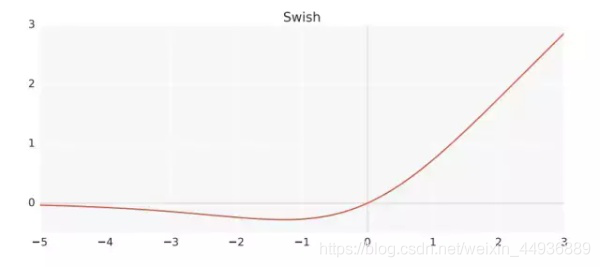s w i s h [ x ] = x × σ ( x ) swish[x]=x×\sigma(x) swish[x]=x×σ(x)，其中 σ ( x ) = s i g m o i d ( x ) = 1 / （ 1 + e − x ） \sigma(x)=sigmoid(x)=1/（1+e^{-x}） σ(x)=sigmoid(x)=1/1+ex

#### 7.1 h-swish 函数：

h h h- s w i s h [ x ] = x R e L U 6 ( x + 3 ) 6 swish[x]=x\frac{ReLU6(x+3)}{6} swish[x]=x6ReLU6(x+3)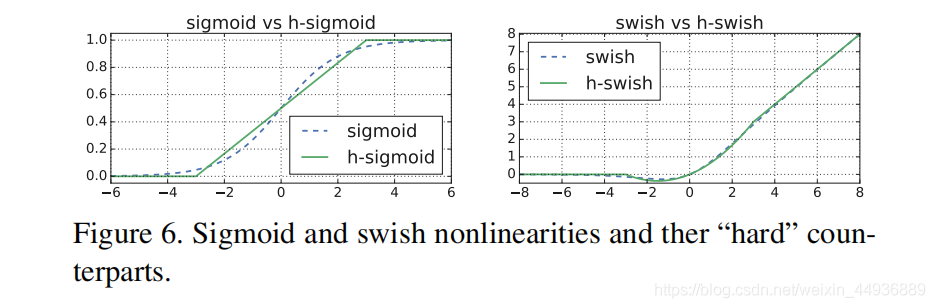### （八）网络结构：

#### 8.1 MobileNetV3-Large：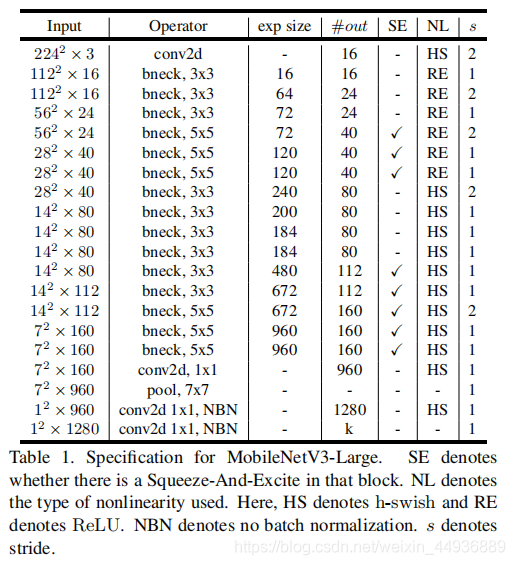#### 8.2 MobileNetV3-Small：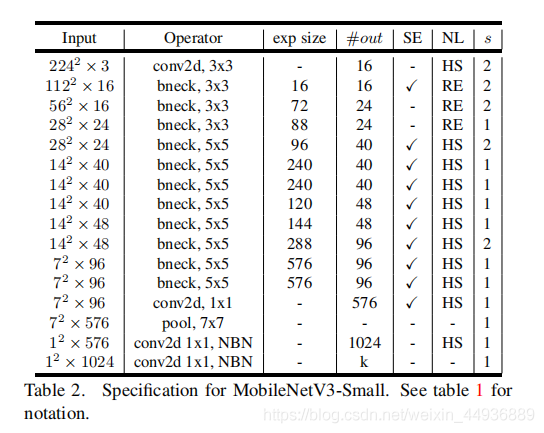###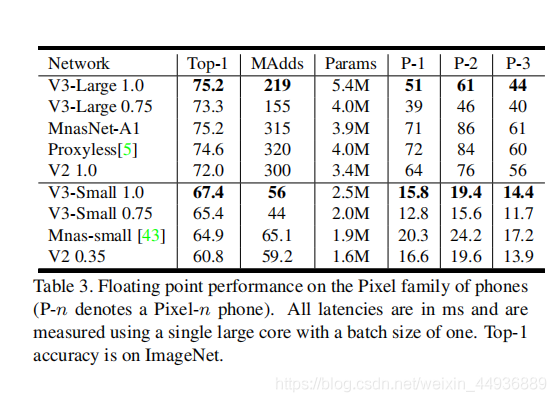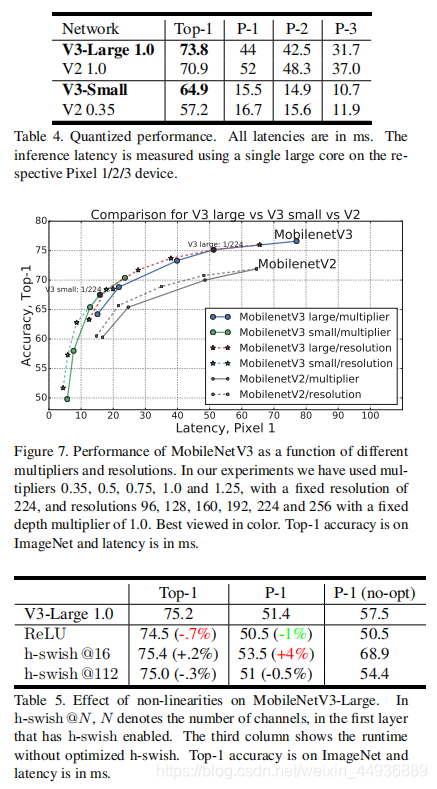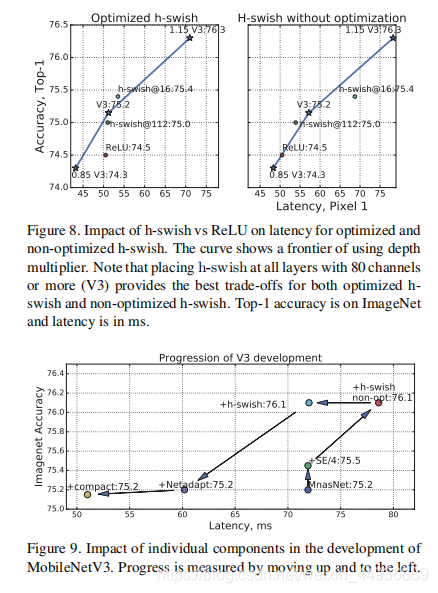# 定义并训练模型
model = pdx.cls.MobileNetV3_small_ssld(num_classes=num_classes)
model.train(num_epochs=2,
train_dataset=train_dataset,
train_batch_size=32,
log_interval_steps=20,
eval_dataset=eval_dataset,
lr_decay_epochs=,
save_interval_epochs=1,
learning_rate=0.01,
save_dir='output/mobilenetv3')

## 五、评估模型性能

save_dir = 'output/mobilenetv3/best_model'
model.evaluate(eval_dataset, batch_size=1, epoch_id=None, return_details=False)
2020-05-18 09:25:35 [INFO]	Model[MobileNetV3_small_ssld] loaded.
2020-05-18 09:25:35 [INFO]	Start to evaluating(total_samples=2243, total_steps=2243)...

100%|██████████| 2243/2243 [00:58<00:00, 38.38it/s]

OrderedDict([('acc1', 0.9790459206419974), ('acc5', 1.0)])# 进行模型量化并保存量化模型
pdx.slim.export_quant_model(model, eval_dataset, save_dir='./quant_mobilenet')
print('done.')
# 加载量化后的模型并进行评估
quant_model.evaluate(eval_dataset, batch_size=1, epoch_id=None, return_details=False)

## 七、总结：

### 在本项目中我们完成了以下任务：

下载安装命令

## CPU版本安装命令

## GPU版本安装命令


## 关于作者：

0
0 收藏

### 作者的其它热门文章0 评论
0 收藏
0